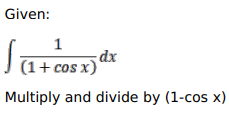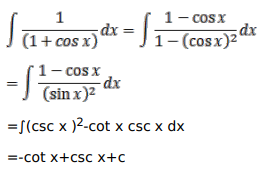# Mark against the correct answer in each of the following:

Question:

Mark $(\sqrt{)}$ against the correct answer in each of the following:

$\int \frac{1}{(1+\cos x)} d x=?$

A. $-\cot x+\operatorname{cosec} x+C$

B. $\cot x-\operatorname{cosec} x+C$

C. $\cot x+\operatorname{cosec} x+C$

D. None of these

Solution: